Home » Math » FREE Subtracting Decimals Activity With Base Ten Blocks

FREE Subtracting Decimals Activity With Base Ten Blocks

Do you have a child struggling with subtracting decimals? This subtracting decimals activity works on regrouping with base ten blocks.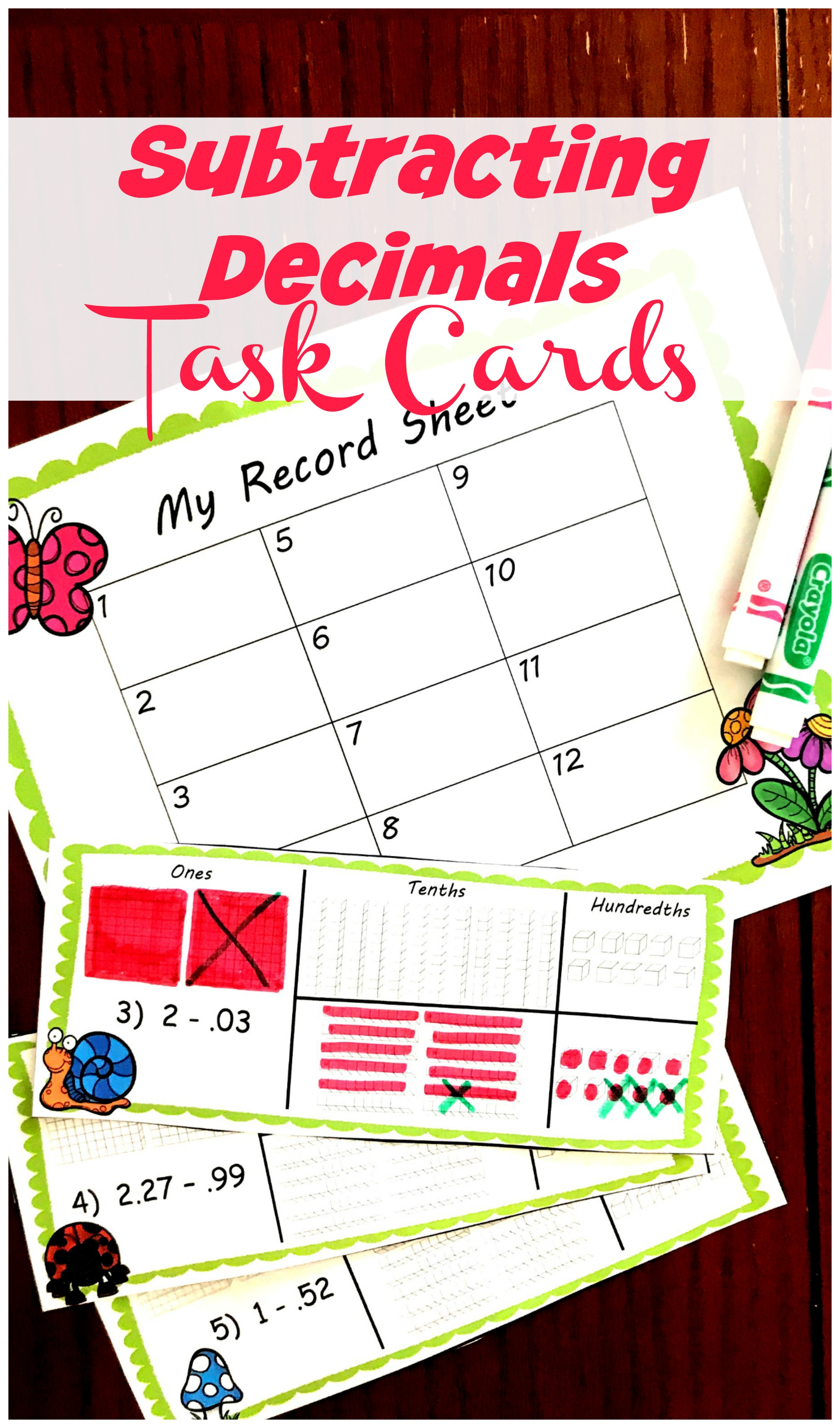Subtracting with regrouping is just plain hard. But when you add decimals…..it gets a little crazier. Subtracting 1 – .56 requires students to have an understanding of the ones, tenths, and hundredths place. Seriously, if they don’t have that understanding how can they even line up an expression like that.

Well today, I have some simple task cards that will help children practice the place value of decimals while subtracting. It is a great subtracting decimal activity for any child, but especially those struggling with subtracting decimals that require regrouping.

If you have a child that does not understand decimals, and what each digit stands for….

This is a great place to start.Prep-Work:

So there are three ways you can prep this activity.

One time use:

For this one, simply print off the cards and provide color pencils. A child can color in the base ten blocks using colored pencils.

Multi Uses (No-Prep)
1. First, print off the task cards on card stock paper, and place them in sheet protectors.
2. Next, print off one record sheet per child using regular paper.
3. Finally, provide dry erase markers and pencils.
Multi Uses (A Little More Prep)
1. Begin, by printing off the task cards on card stock paper and then cutting them out.
2. Next, laminate them, hole punch a hole in the top left corner, and use a ring to hold them together.
3. Next, print off one record sheet per child using regular paper.
4. Finally, provide dry erase markers and pencils.

Subtracting Decimal Activity

Before you begin this activity, I highly suggest using real base ten blocks and then move into the task cards. It may help children understand what they are doing if the physically use the materials before just coloring them in.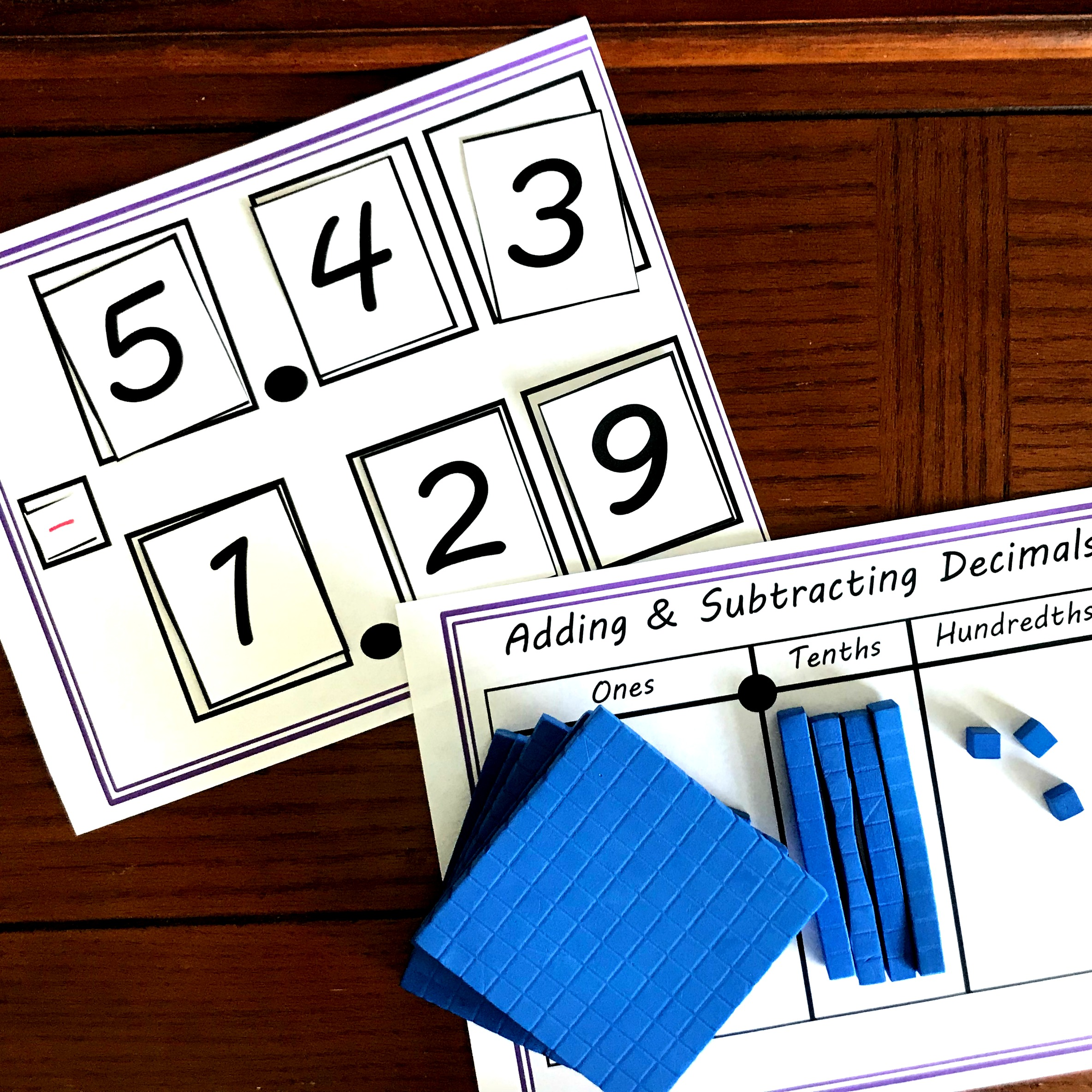Let’s look at how to use one of these cards.

The first step is to look at the first number in the expression, and color in that amount.Next, we need to cross off the amount the second number in the expression represents.

In this example we can’t do that, so we must do some regrouping.

I begin, by crossing off the flat in the one’s place, and then coloring in ten longs in the tenths place.Next, I need to cross off a long, and color in ten cubes in the hundredths place.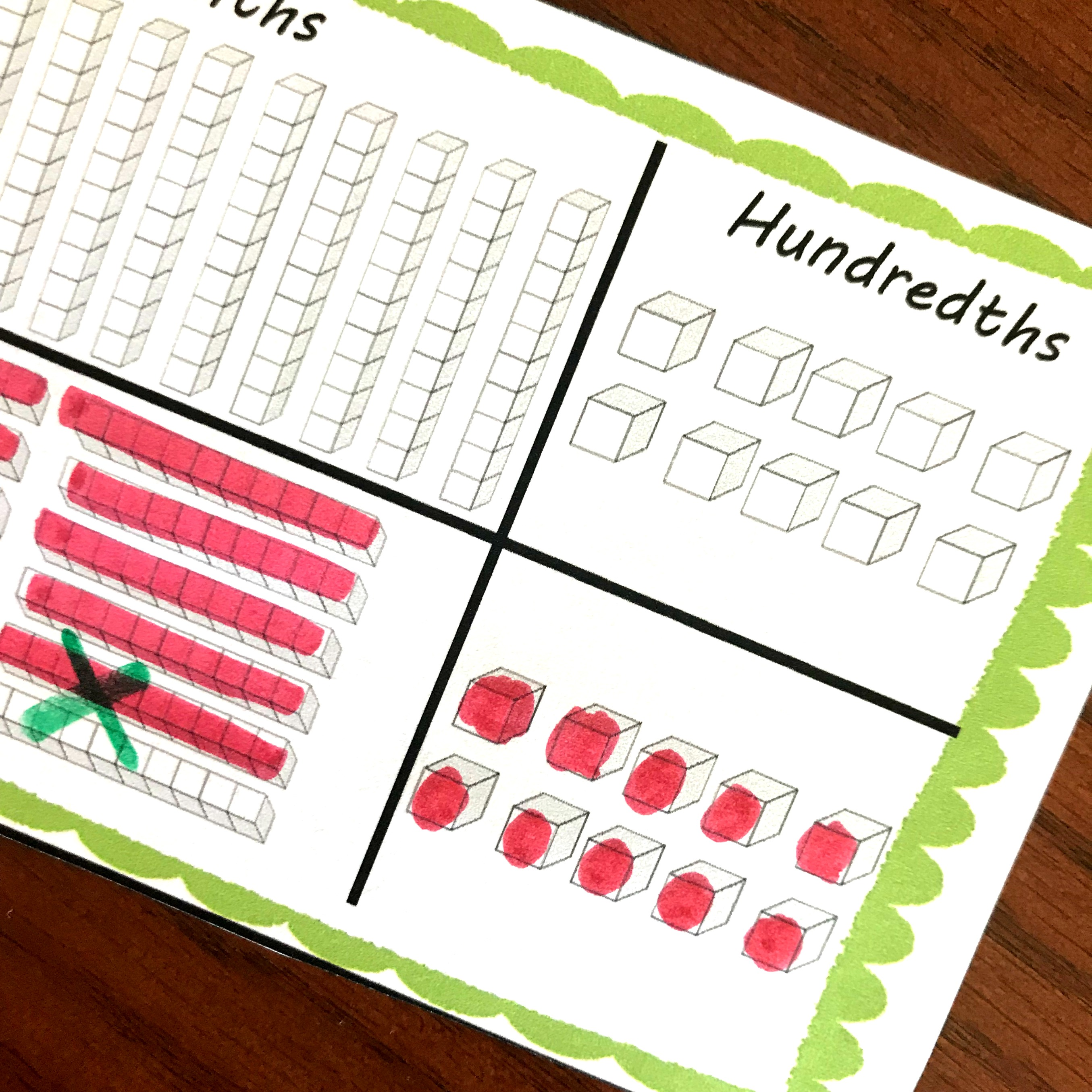Now that all the regrouping is done, I can subtract. I cross off three hundredths which is three cubes.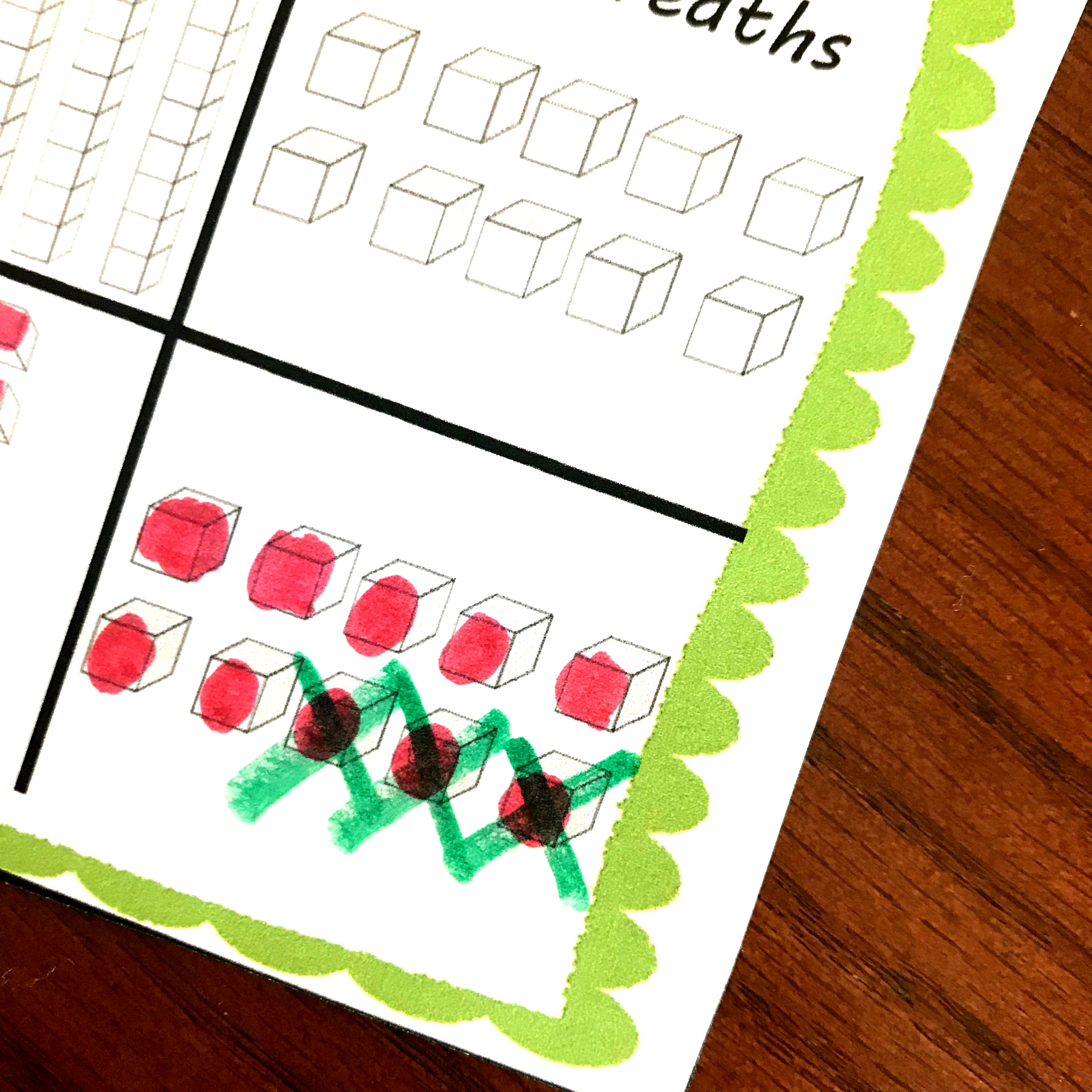Finally, we count up what is left. There is one whole, 9 tenths, and seven hundredths. Soooo the answer is 1.97!!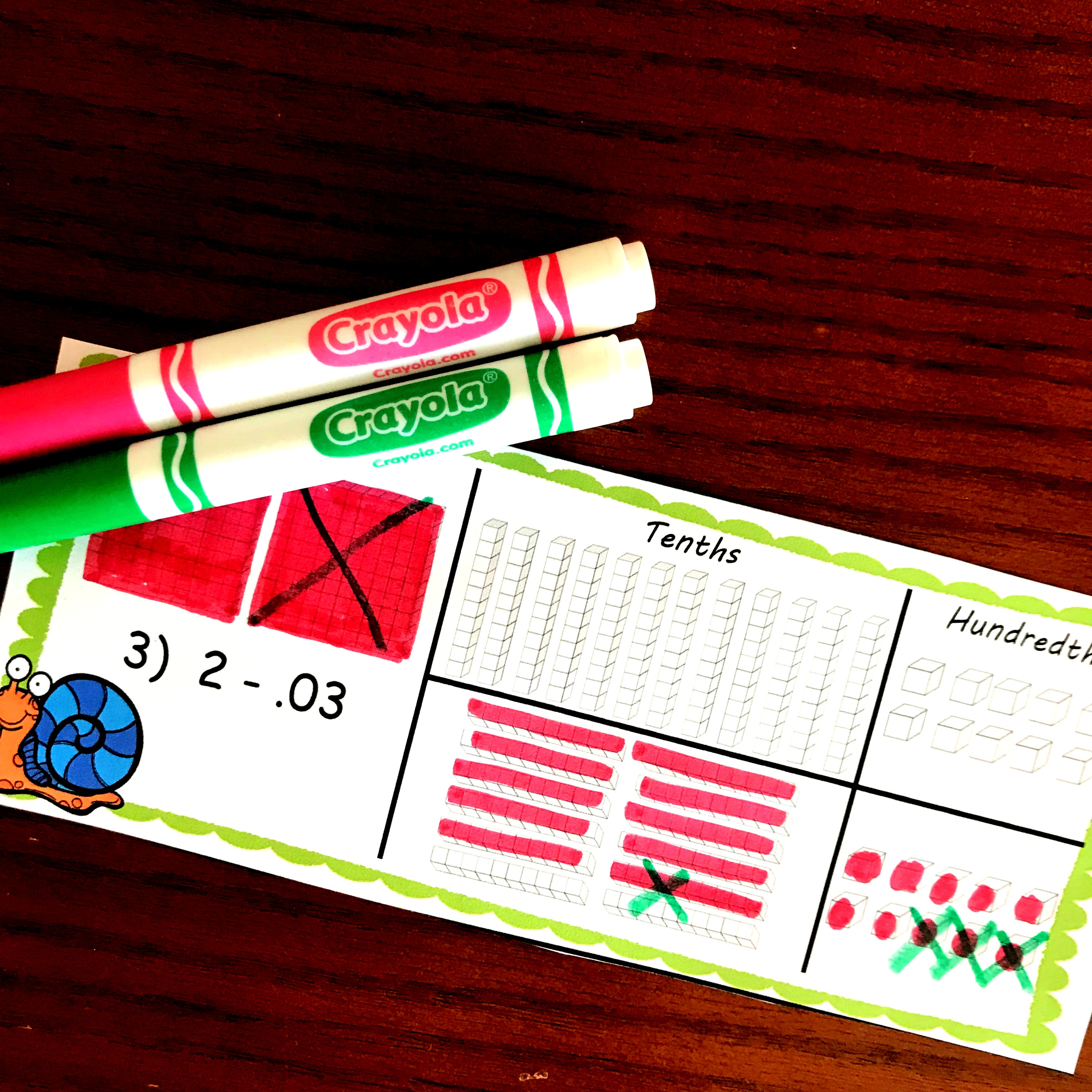I hope these help your children understand place value a little more, and give them a good visual for what is happening when I subtract decimals.

You’ve Got This,

Rachel